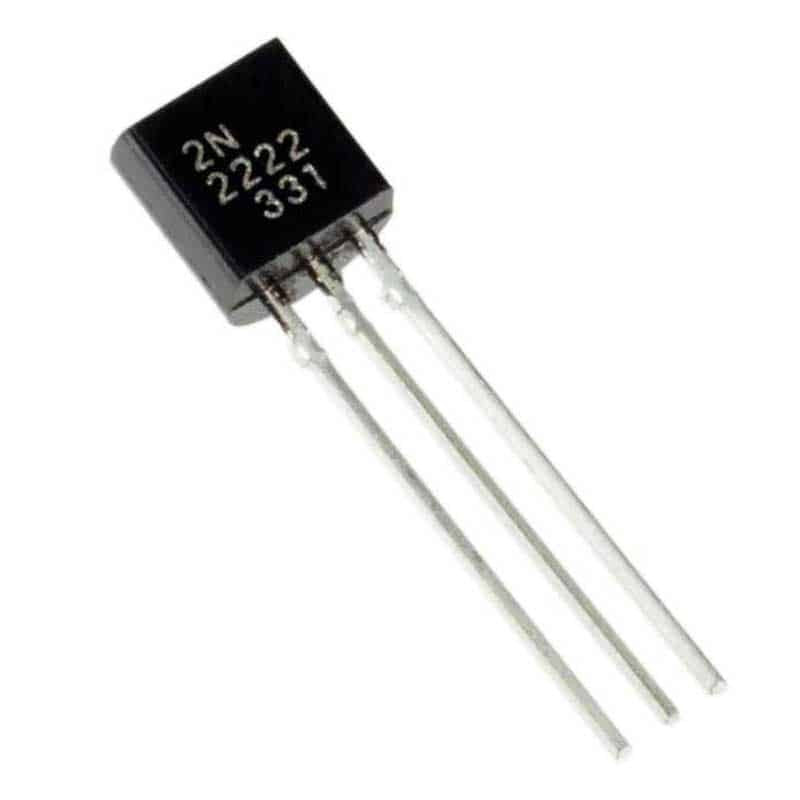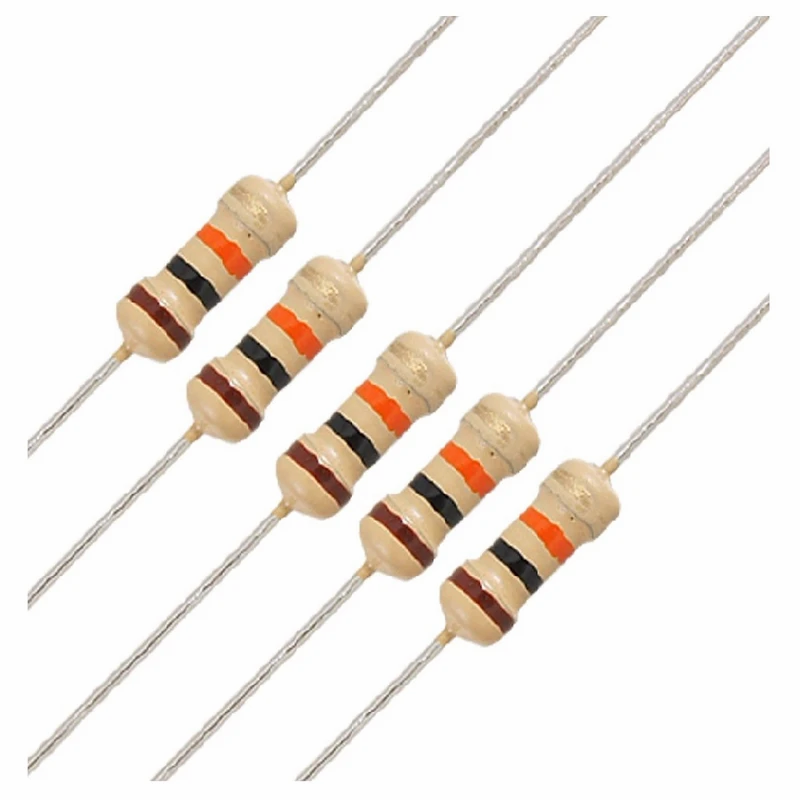• LearnElectronics

# EX-OR gate using transistor

Updated: May 3

The EX-OR stands for the Exclusive–OR Gate, also abbreviated as #XOR Logic Gate. The output of an EX-OR Gate is HIGH only when either of the two inputs is HIGH and is LOW when both the inputs are identical.

We can represent the Boolean expression of the EX-OR Logic Gate as output -

Q = A.B' + A'B or (A + B).(A' + B') or A B

The below image shows the symbolic representation of the EX-OR Gate: -XOR-Gate

The truth table of XOR Gate is given below.

```INPUT A          INPUT B          OUTPUT(Y)
0  		    0			0
0		    1			1
1		    0			1
1		    1			0```

Exclusive (xor) logic gates can be constructed from other logic gates as shown below.XOR Gate using other Logic Gates

The above figure shows XOR Gate built using NAND, OR, and AND Gates.

The inputs pass through #NAND and #OR Gates simultaneously which generate corresponding outputs which are then together given as an input to the #AND gate. The expression can be derived as follows.

For NAND Gate,

Input: A, B Output: (A . B)' = A' + B'

For OR Gate,

Input: A, B Output: A + B

For AND Gate,

Input: A'+ B', A + B Output: (A'+B') . (A + B)

= A'.A + A'.B + B'.A + B'B

= 1 + A'.B + B'.A + 1 ( Rule: P.P' = 1 )

= A'.B + A.B' (Rule: 1 + P = P)

= A'B + AB' (XOR Output)

## Components Required

### 1. 6 Transistors2N222A Transistor

It is a low-power amplifying transistor. A common NPN Bipolar Junction transistor (#BJT). This transistor is usually used for Switching purposes as we have used it in our circuit. It has a Base, Emitter, and Collector Terminal, the notations to indicate the same will be given on the Transistor itself.

### 2. LEDLED

Light Emitting Diode is a commonly used light source. It is a semiconductor that emits light when current flows through it.

### 3. Power Supply ModulePower Supply Module

This #module is specially used for providing voltage supply to circuits designed with components on a small scale. The module has a Barrel jack the can be connected to the DC supply directly. The Voltage is regulated to supply 6-12 V DC. This regulated voltage is then supplied to the circuit built.

The power channel can be configured to supply 3.3V, 0V, or 5V. It has a control button that can be operated as per our requirement to start and stop the power supply. It has an onboard LED which indicates when the power is supplied through the module. For this circuit, the power supply can be 5V or 6V.

### 4. Resistors10k Ohm Resistor4.7k Ohm Resistor

Resistors are passive devices that restrict the flow of current or divide the voltage through the circuit. The resistors used for this circuit are 10k Ohm and 4.7k Ohm resistor. 10k Ohm Resistor is connected before the transistor, i.e. the input power passes through these resistors and then to the transistors. The 4.7k Ohm resistor is connected before the LED so that a lesser amount of voltage is diverted to the LED.The breadboard is the basic component of any circuit building process. All components, be it input sensors or output display devices are connected to the power supply, microcontroller using wired connections through a breadboard. The holes in the breadboard are in series. There are various sizes like full-sized, half-sized, and mini breadboards.

In this circuit, as we have several connections, two breadboards can be used to make the circuit less complex and understandable. One of the breadboards can be used to configure the Ground and Voltage terminal through which connections can be made to the different components on the Full Breadboard.

### 6. Jumper WiresJumper wires

These are the main components that are used to establish the connections between different devices of the circuit.

## XOR gate using transistor-Circuit ConnectionsCircuit Connections

In the circuit, we have six #transistors the two transistors (T1 and T2) form the NAND gate, the two transistors (T3 and T4) form OR gate, the two transistors (T5 and T6) form the AND gate. There are two inputs A and B. Input A is connected to the base of transistors T1 and T3, Input B is connected to the base of transistorsT2 and T4. Transistors T1 and T3 are connected in series i.e., the emitter of transistor T1 is connected to the collector of transistor T2.

The power supply of +5V is connected to transistor T1 using a 1K ohm resistor and the emitter terminal of transistor T2 is grounded. The output of transistor T1 is given as input to transistor T5. Transistors T3 and T4 are connected in parallel to each other. The power supply of +5V is connected to the collector terminal of both the transistors T3 and T5. The emitter terminal of transistor T3 grounded using a 5K Ohm resistor. The output from transistors T3 and T4 is given as input of the transistor T6. Transistors T5 and T6 are connected in series. The power supply of +5V is connected to transistor T5. The output is taken across transistor T6.

## Working of XOR gate using transistor

The working of the circuit can be explained using 4 cases according to the truth table. Let us consider each case one by one and understand its working.

### Case 1: Input A = 0 and B = 0When both the inputs A and B are zeroes all the transistors will be in OFF state. The inputs to transistors T5 and T6 are 1 and 0 respectively. The transistors T5 and T6 form an AND and one of the inputs to the AND is LOW so the output will be LOW and thus the overall output of the circuit will be LOW and the LED will be in an OFF state indicating the output of the EX-OR gate using a transistor LOW i.e., 0.

### Case 2: Input A = 0 and B = 1When Input A = 0 and B = 1 transistors T2 and T3 will be in OFF state, transistors T1 and T4 will be in ON state. The inputs to transistors T5 and T6 are 1 and 1 respectively. The transistors T5 and T6 form an AND and both the inputs to the AND are high so the output will be high and thus the overall output of the circuit will be HIGH and the LED glows indicating the output of the EX-OR gate using a transistor as HIGH i.e., 1.

### Case 3: Input A = 1 and B = 0When Input A = 1 and B = 0 transistors T2 and T3 will be in ON state, transistors T1 and T4 will be in OFF state. The inputs to the transistors T5 and T6 are 1 and 1 respectively. The transistors T5 and T6 form an AND and both the inputs to the AND are high so the output will be high and thus the overall output of the circuit will be HIGH and the LED glows indicating the output of the EX-OR gate using a transistor as HIGH i.e., 1.

### Case 4: Input A = 1 and B = 1When Input A = 1 and B = 1 all transistors T1, T2, T3, and T4 will be in ON state. The inputs to the transistors T5 and T6 are 0 and 1 respectively. The transistors T5 and T6 form an AND and one of the inputs to the AND is LOW so the output will be LOW and thus the overall output of the circuit will be LOW and the LED will be in an OFF state indicating the output of the EX-OR gate using a transistor LOW i.e., 0.

Watch the complete video and check out the practical implementation